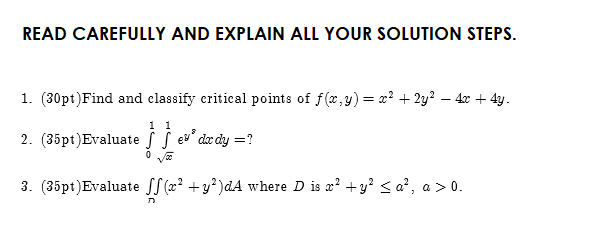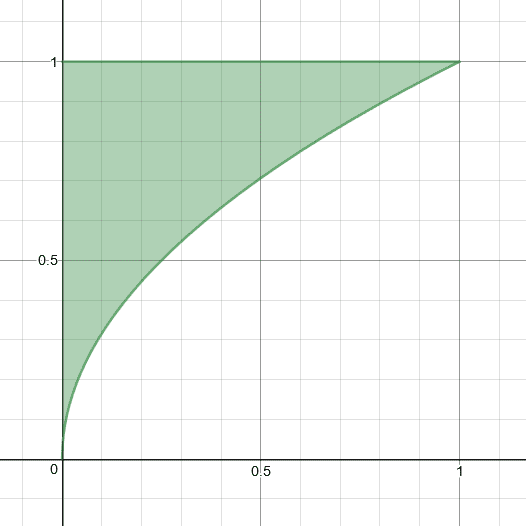# My Homework - Can You Help Me?

• MHB
ozgunozgur
I am trying to solve these questions for hours :/Last edited by a moderator:

Gold Member
MHB
Let's begin with the first problem...what have you tried so far?

Gold Member
MHB
Yes, I agree with your finding of a minimum at $$(2,-1)$$. It is a global minimum. For the second problem, isn't the integrand:

$$\displaystyle e^{y^8}$$ ?

ozgunozgur
Yes, I agree with your finding of a minimum at $$(2,-1)$$. It is a global minimum. For the second problem, isn't the integrand:

$$\displaystyle e^{y^8}$$ ?
Ah sorry. My third question is true? And second is a bit problem.

ozgunozgur
Yes, I agree with your finding of a minimum at $$(2,-1)$$. It is a global minimum. For the second problem, isn't the integrand:

$$\displaystyle e^{y^8}$$ ?
Isn't it gamma function for 8y?

ozgunozgur
Please help me for second question, I guess I have to draw a sketch. Can you draw it full?

Gold Member
MHB
The use of exponential integral and gamma functions goes beyond what I was taught in Calc 2. We did multi-variable calculus in Calc 3, but still worked with elementary functions. I can't help you with this problem. Perhaps someone else can.

Homework Helper
MHB
Questions 1 and 3 are fairly straight forward applications of multivariable Calculus with elementary functions.
So I think question 2 must also be such a straight forward application.
Looks to me as if the question should read:
$$\int_0^1 \int_{\sqrt x}^1 \exp(y^3)\,dy\,dx=\,?$$
That is, with power $3$, and with the variables of integration swapped.
Now we can solve it by swapping the order of integration, which is likely intended. And yes, a graph may help.

ozgunozgur
Questions 1 and 3 are fairly straight forward applications of multivariable Calculus with elementary functions.
So I think question 2 must also be such a straight forward application.
Looks to me as if the question should read:
$$\int_0^1 \int_{\sqrt x}^1 \exp(y^3)\,dy\,dx=\,?$$
That is, with power $3$, and with the variables of integration swapped.
Now we can solve it by swapping the order of integration, which is likely intended. And yes, a graph may help.

Homework Helper
MHB
Please show an attempt to swap the order of integration.
Or otherwise give us a clue in some detail where you are stuck.
You should have an example in your textbook that shows how it is done.
If you can't find such an example, you might take a look at this example.

ozgunozgur
Please show an attempt to swap the order of integration.
Or otherwise give us a clue in some detail where you are stuck.
You should have an example in your textbook that shows how it is done.
If you can't find such an example, you might take a look at this example.

#### Attachments

Gold Member
MHB
Now that Klaas has figured out what #2 is actually supposed to be, let's look first at the region over which we are to integrate:Reversing the order of integration, we may write:

$$\displaystyle I=\int_0^1\int_0^{y^2} e^{y^3}\,dx\,dy$$

Can you proceed?

Homework Helper
MHB
For question 3 we should use a switch to polar coordinates:
\begin{tikzpicture}
\filldraw[green!70,draw=gray] (0,0) circle (2.5);
\draw[help lines] (-3.2,-3.2) grid (3.2,3.2);
\draw[->] (-3.4,0) -- (3.4,0);
\draw[->] (0,-3.2) -- (0,3.2);
\draw foreach \i/\x in {-2.5/-a,2.5/a} { (\i,0) node[below] {$\x$} };
\draw foreach \i/\y in {-2.5/-a,2.5/a} { (0,\i) node[ left ] {$\y$} };
\end{tikzpicture}
$\iint_D (x^2+y^2)\,dA = \int_0^a \int_0^{2\pi} r^2 \cdot r\,d\theta \,dr$

ozgunozgur
Thank you very much.
https://ibb.co/0XxGrDr
https://ibb.co/ZKNtgZy
I rearranged I wonder if I wrote extra or are we going right?

ozgunozgur
ozgunozgur
I was sent the other solut. Can you edit these two solving. https://ibb.co/c6S7r8n

ozgunozgur